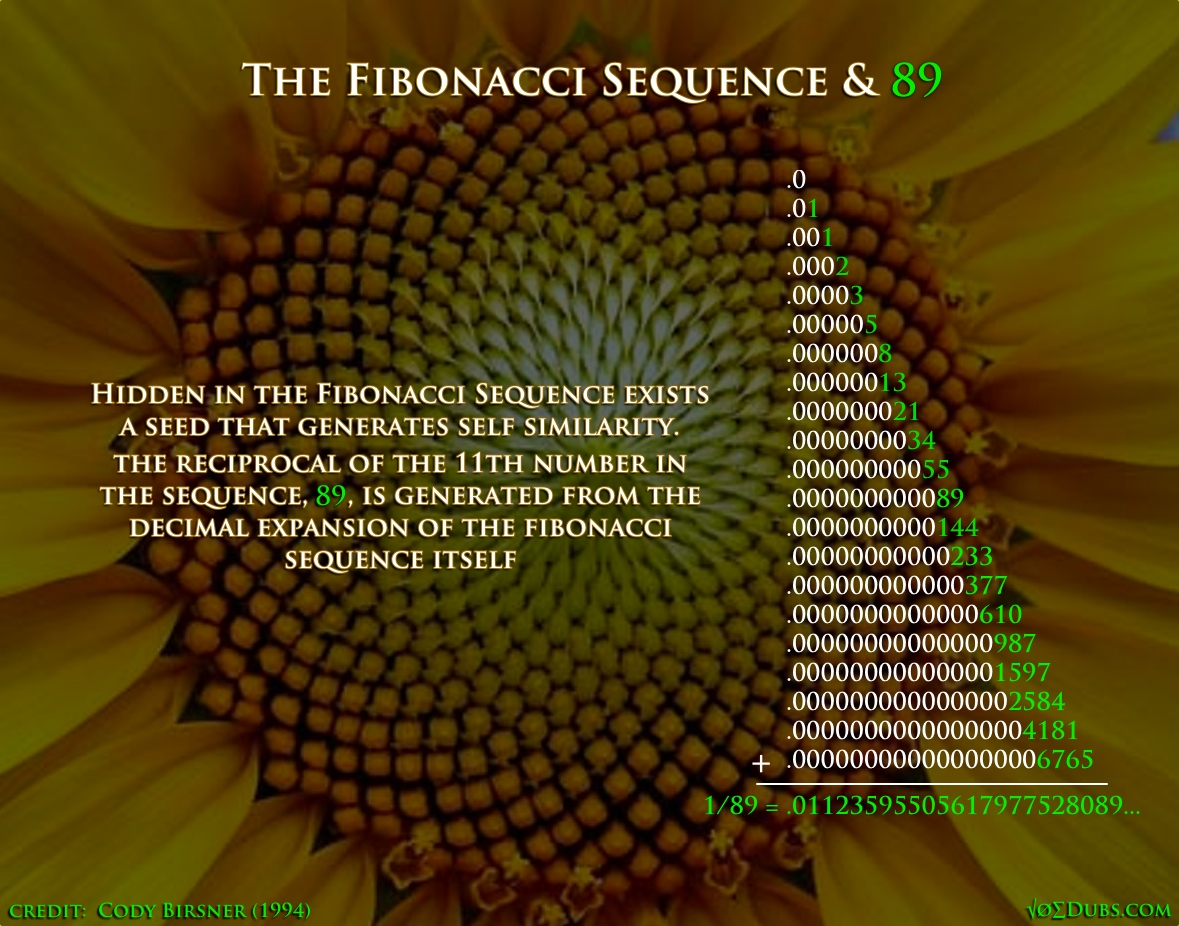The numbers 1 through 12 are what’s called the Dodecad.  Do means 2.  Decad means 10. These are not just numbers, but qualities of reality and the numerical armature that nature uses to build herself.  There’s twelve hours on the clock, twelve inches in a foot, and twelve signs of the Zodiac.  Have these measures any correlation? Is it all just a big coincidence? “There are too many coincidences for it to be all just coincidence” – unknown This is 99.99% accurate according to NASA in 2014.  Now, NASA has been known to lie… Perhaps they are just playing a trick on us? Who knows! Some days I think they might knock on my door and congratulate me on recognizing their cosmo canonical number prank. The dodecahedron is the most mysterious of the Platonic solids.  Even mentioning this archetypal form outside the walls of Plato’s academy…

1

## Fibonacci: The Fractions of LifeThe Fibonacci Sequence is Nature’s favorite series of numbers.  They are found wherever there is life.  Closely associated with phi, this sequence actually generates this golden ratio when any number is divided by the number before it. Phi = 1.618033988.. The Fibonacci Sequence: 0, 1, 1, 2, 3, 5, 8, 13, 21, 34, 55, 89, 144, 233, 377, 610, 1597, 2584, 4181, 6765, 10946, 17711, 28657, 46368, … Phi and phee are generated from the Fibonacci sequence in this way.  This might be an indication that fractions are important, especially in relation to the Fibonacci numbers, since they create the golden ratio or the divine proportion. The 11th number in the sequence is 89.  Zero is not a number but a placeholder for numeric expansion and contraction.  Interestingly 89 is 11 less than one hundred, but even more interesting is its reciprocal, which is 1/89.…

3

## The Golden Geometry of PhiIt’s important to understand where the most ubiquitous ratio in nature is found by using simple geometry.  The golden geometry of phi.  The divine proportion (the golden mean, Phi, the golden number, Fibonacci’s number) creates fractal expansion and contraction which creates life as we know it. The square root of five can sound scary, but when it is visually represented it’s not as frightening.  The square root of 5 (the cross section of a double square) is essential in the formula for Phi. It’s encoded everywhere from our dna to the geometry of a nautilus shell to the milky way.  Phi is found in plants though phyllotaxis,  or the way the leaves arrange themselves around the stem.  They rotate in golden angles. 222.5 degrees is golden among the 360. “The harmony of the world is made manifest in Form and Number, and the heart and soul…

3

## Synergistic Mathemagics in the Solar SystemThe way we measure space and time is based on a synergistic and mathemagical canon of number that’s intrinsic to our solar system as well as the proportions of man.  As above so below. It just so happens that the Moon’s diameter is almost exactly 2160 miles (99.99+%), the same amount of degrees on the surface of a cube.  Earth’s circumference at the equator is almost exactly 21,600 nautical miles. (99.9+%) The Earth and Moon ‘square the circle‘ Square the Circle: The Moon is the perfect size to solve the riddle. It’s huge in fact, larger than any other in proportion to its planet. Some say we have a double planet system. The Moon is 27.3% the size of Earth. It also happens to take 27.3 days to complete an orbit around Earth. Need I point out the fact that the Moon influences our emotions. Women’s menstruation cycles last…

1